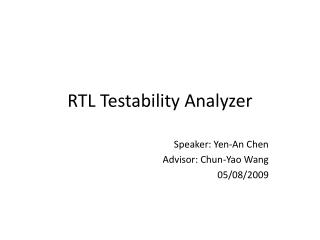# RTL Testability Analyzer - PowerPoint PPT PresentationDownload PresentationRTL Testability Analyzer

RTL Testability AnalyzerDownload Presentation## RTL Testability Analyzer

- - - - - - - - - - - - - - - - - - - - - - - - - - - E N D - - - - - - - - - - - - - - - - - - - - - - - - - - -
##### Presentation Transcript

1. RTL Testability Analyzer Speaker: Yen-An Chen Advisor: Chun-Yao Wang 05/08/2009

2. Outline • Introduction • Flow chart • THLF structure • Fault detective probability • Experiments • Discussion • Future work

3. Introduction • We want to estimate testability at RTL level instead of calculating testability at gate level, so that we can eliminate the time of synthesis. • We want to use high-level synthesis to do the simulation instead of simulating gate-level netlist. • Fault detection probability: to show the difficulty to simultaneously set a value to a node from the PIs and observe the node in the POs.

4. Flow Chart RTL behavior Read RTL behavioral code first. After using heigh-level synthesis, we got THLF. And then we use Monte Carlo method on THLF netlist to do the simulation. Finally, we can get the testability of statements in each blocks. High-level Synthesis Levelizer THLF • Levelizer includes: • Levelize • Manipulate OR gates Monte Carlo Testability Report

5. THLF structure Data part Decision point Control unit

6. Fault detection probability • SBATA deals with AND, OR, NOT gates. • Besides these three gates, we also want to handle XOR gates. AND gate: Cr(wk) = 1101 wk XOR gate: Cr(wk) = 1101 wk

7. Experiments • Three types of cases and difficulties: • Case1: only logic gates. • Case2: with conditions • Case3: with hierarchical elements.

8. Case1: RTL Behavior: Synthesis: Full_Adder: module fulladder(a, b, cin, s, cout); input a; input b; input cin; output s; output cout; reg s, cout; always @ (a or b or cin) begin s = a ^ b ^ cin; cout = a&b | a&cin | b&cin; end endmodule 1 THLF: Statement tree

9. Monte Carlo (Simulation) Synthesis: THLF: Result: I can calculate the same fault detective probabilities with gate-level netlist. But how cout I collect these values to statement 1?

10. Case2: RTL Behavior: Synthesis: module test2(A, B, con, D, E); input A, B, con; output D, E; wire A, B, con; reg C, E, D; always @ (A or B or con) begin C = A; if(con) D = C; else D = B; E = B; end endmodule 1 THLF: 2 Statement tree 3 4

11. Monte Carlo (Simulation) Simulate gate-level netlist: 0.5 0.5 0.5 0011 0011 0011 0011 0.5 0111 0011 0 0.5 0 0101 0101 0101 0000 1000 0.5 0111 0 0.25 0101 0.75 0111 0111

12. Monte Carlo (Simulation) Simulate THLF netlist: 0111 0011 0011 1111 0011 0011 0011 0111 0111 0101 0111 1000 1111

13. Monte Carlo (Simulation) 0.5 0.5 0.5 0011 0.5 0.5 0.5 0 0.5 0 0101 0111 0.5 0.5 Result: I can calculate almost the same fault detective probabilities with gate-level netlist.

14. Case3: RTL Behavior: Synthesis: 1. The netlist may be different by different libraries “+” uses. 2. How to find the critical vector of “+” ? Calculate cout: module counter(a, cout); input a; output cout; regcout; always @ (a) begin cout = a + 1; end endmodule 1 THLF: Statement tree

15. Discussion • How to find the critical vector of those wires passing through non-logic gates? • How to collect the fault detective probabilities we calculate? • What information should I take in to consideration? • How to verify whether the fault detective probabilities are precise or not? • What conclusion can I guarantee?

16. Future Work • Keep experimenting and thinking or finding more information.